# iFit: Models Phonons (fit models)

## Phonon dispersion from ab-initio force estimate and dynamical matrix

The sqw_phonons model computes phonon dispersions (lattice dynamics) using the Atomic Simulation Environment (ASE) in the so-called small displacement methodology. The only needed input for the model to run is the name of a configuration file describing the material lattice and atom positions. This file can be in any ASE supported format, e.g. POSCAR, CIF, PDB, ... This model requires to install external software on the computer. See Requirements (and Installation) below.

This documentation lists all possible options.

 S(q,w) Description Dimensionality Parameters sqw_phononsPhonon dispersions from the Dynamical matrix, using forces estimated by ab-initio using ASE and a selection of DFT codes (EMT, GPAW, ABINIT, Elk, QuantumEspresso, VASP). 4D (HKLw)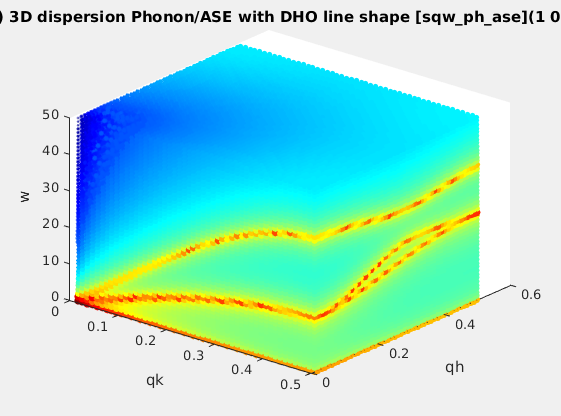Creation: POSCAR, CIF, PDB, ... Then, only the DHO line shape. ab-initio implies no (few) tunable parameter. Axes in rlu.

The procedure is entirely automatic. A supercell is automatically generated, then forces are estimated by differentiation of a set of atom displacements. Temporary data files are created. The model can then be evaluated onto an HKLE trajectory, and plotted.

### A few words about the theory

Let us consider a perfect crystal, at very low temperature, in the harmonic approximation close to its equilibrium. The dynamical matrix D is defined as:
Dαβ=1/√(MαMβ) ∂2V/(∂uα∂uβ) where V is the inter-atomic potential, M is the atom mass, αβ are atom indices, u is the displacement of these atoms around their equilibrium position.
The equation of motions is then:
d2uα/dt2 = - ∑ Dαβ.uβ
In order to simplify this expression, we assume a periodic motion:
uα=Uα(k) e i(k.r - ωt)
where r is the position of the atom in a so-called super-cell, which replicates the lattice primitive cell, and its extent sets the potential extension distance, which is assumed to vanish at long distance. k and ω are the momentum and energy of the wave.

The methodology is then to displace each atom by a small quantity, then compute the Hellmann-Feynman force F acting on the atom to restore the equilibrium. This step is commonly achieved by an ab-initio code such as VASP, ABINIT and QuantumEspresso. Then the potential partials 2V/(∂uα∂uβ) are estimated by differentiating the forces as F = -∇V after displacing atoms by small quantities. Finally, the dynamical matrix is computed for each desired wave-vector k, and its eigen-modes are extracted. Currently, there are a few packages which do perform the dynamical matrix estimate and diagonalisation steps, among which PHON, PHONON, ASE and PhonoPy. We here use ASE together with PhonoPy (when available).

In addition, once the phonon spectrum is known, we can compute the coherent vibrational density of states g(ω) as, for each energy ω, the number of phonon modes lying within a frequency [ω-dω : ω+dω] for all wavevectors. The density of states is normalised to the total number of modes:
∫g(ω) dω = 3N

The neutron scattering intensity of the vibrational modes is evaluated as (with a damped harmonic oscillator line shape)

I(Q,ω) ~ Amplitude * |F(Q)|2 DHO(ω0,Γ) [n(ω)+1] / ω0
|F(Q)|
2 = | ∑ bcoh e -W(Q)|Q.e|2 e-iQ.r |2

where e is the phonon mode polarisation vector (multiplied by √mass), ω0 is the mode normal frequency, bcoh is the coherent neutron scattering length, W(Q) is the Debye-Waller function, and n(ω) is the Bose factor (population). The DHO line shape will be centered around the energy Ω2 = ω2 + Γ2 and Γ is the mode damping, which holds the imaginary part of the phonon frequency (when becoming unstable/negative).

The Debye-Waller function is estimated as:

W(Q) = |Q.e|2 [2n(ω0)+1] /0

The form factor evolves roughly as e-1/2 <u^2>Q^2 |Q|2 which  indicates that at low and large Q, the phonon modes are not intense. In the first Brillouin zone (e.g. HKL < 0.5), the transverse modes are not measurable with neutrons as |Q.e| is null when the transverse polarisation vector e is perpendicular to Q. The Amplitude parameter includes the incoming neutron flux I0, detector efficiency, etc...

Amplitude ~ I0 kf/ki

where kf and ki are the final and incident neutron wavevectors.

### Syntax

The simplest syntax to create the model is to call: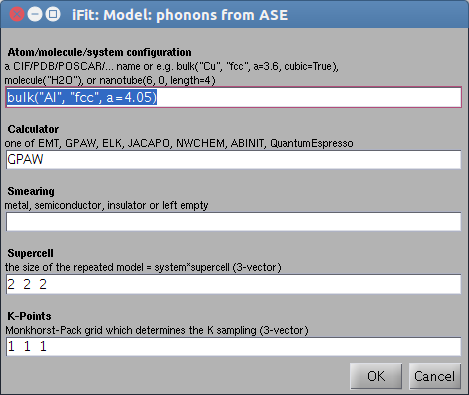s = sqw_phonons;        % displays an entry dialog box and proceeds with a fully automatic computation, and plots final results. With QuantumEspresso, a dialog may show up to select pseudo-potentials when there is more than one possibility.
The general syntax for creating  the S(q,w) model is any of:
s = sqw_phonons('defaults');        % creates a simple fcc Al model and QE as calculator
s = sqw_phonons(configuration);
s = sqw_phonons(configuration, options, ...);

s = sqw_phonons(configuration, calculator, options, ...);
The result is a 4D S(hkl,w) phonon dispersion Model (iFunc object).

You may as well re-import a previous calculation from its directory, with:
s = sqw_phonons(directory);
Such a directory may contain a POSCAR* file, any previous lattice definition (atoms.pkl as an ASE Atoms object), phonon and vibrational calculation steps, as well as PhonoPy FORCE_SETS and yaml files.

In addition, a web based interface can be set up very easily by installing, on a Debian-type system, the ifit-web-services package, and then browse to e.g. http://localhost/ifit-web-services.

Once created
, we recommend to save the model with:
>> save 'my_model' model
You can later load this model again within iFit, without the need to recompute forces (which may be a long process with DFT codes).

The model parameters, once built, are the following:

p(  1)=  Amplitude
p(  2)=  Gamma  Damped Harmonic Oscillator width in energy [meV]
p(  3)=  Background
p(  4)=  Temperature  [K] used to compute the Bose factor n(w)
p(  5)=  Energy_scaling
The axes needed for the evaluation are expressed in rlu for QH,QK,QL and in meV for the energy [1 meV = 241.8 GHz = 11.604 K = 0.0965 kJ/mol]. The model can be evaluated by giving axes and model parameters (see examples below).
• When all axes are given as vectors of same orientation and same length, the HKLE set is assumed to be list of 'events'.
• When the H K L axes are vectors of same length/orientation, the HKL locations is assumed to be a q-path in the BZ, but the E (energy) axis can be of different length/orientation to compute the Model along (q,E) path, resulting in a 2D object. Typically, the band_structure function does this, to provide the phonon dispersion curve along principal directions.
• When the H K L E axes are given as mixed orientations/length, as vectors or matrices, the Model evaluation is done on a HKLE cube, which is well suited to visualize the whole dispersion surfaces. In the example below, ql has a different orientation (it is transposed) as qh and qk, which triggers a 4D HKLE cube evaluation.
• When the qh,qk,ql axes span over a whole Brillouin zone, e.g. [-0.5 : 0.5], the phonon density of states (DOS) is computed, and stored in s.UserData.DOS, as well as partial DOS in s.UserData.DOS_partials (per mode).
• The maximum phonon energy is stored as s.UserData.maxFreq
• You may evaluate the powder dispersion using the powder(s) call.
• The thermo-chemistry quantities can be obtained with the thermochemistry(s) call.
The resulting iData object can then be plotted, such as in the following example (grid max energy 50 meV):
```	>> qh=linspace(0.01,.5,50);qk=qh; ql=qh; w=linspace(0.01,50,51); 	>> f=iData(s,[],qh,qk,ql',w); 		% evaluate model into an iData. 	>> plot3(log(f(1,:,:,:)));		% plot QH=0 plane
```

### The system configuration (atoms, unit cell)

The configuration is the location of any file format supported by ASE, which describes the system geometry (atom types and positions in the cell). In addition, the system configuration can be given as a bulk, molecule, crystal and nano-tube constructors from ASE.

 configuration Description a file name [ ifitpath 'Data/POSCAR_Al'] Any file in a format supported by ASE, including CIF, ETSF, Gromacs, LAMMPS, PDB, SHELX, VASP/POSCAR, MaterialStudio, XYZ. See full list. You can use as example (provided in iFit Data directory): POSCAR_Al POSCAR_AlN POSCAR_MgO POSCAR_Si POSCAR_NaCl POSCAR_SrTiO3 a COD ID 'cod: 1512124' It is possible to retrieve a Crystallography Open Database (COD) entry by specifying its COD ID. This feature requires a valid network connection. See more details here. a chemical formula 'cod: O3 Sr Ti' It is possible to retrieve a Crystallography Open Database (COD) entry by specifying its chemical formula, in Hill notation, i.e C and H first, then other elements in alphabetical order. A search is made on COD, and when multiple matches are found, a dialogue box pops-up to choose a structure from a list. This feature requires a valid network connection. See more details here. 'bulk("Cu", "fcc", a=3.6, cubic=True)' A bulk material, with formula (Cu, MgO, NaCl), lattice constant and structure (sc, fcc, bcc, hcp, diamond, zincblende, rocksalt, cesiumchloride, fluorite or wurtzite). See description of bulk. `bulk("Cu", "fcc", a=3.6)` 'molecule("isobutane")' Any molecule known to ASE, e.g. 'isobutene', 'CH3CH2OH', 'CH3COOH', 'COF2', 'CH3NO2', 'CF3CN', 'CH3OH', 'CCH', 'CH3CH2NH2', 'PH3', 'Si2H6', 'O3', 'O2', 'BCl3', 'CH2_s1A1d', 'Be', 'H2CCl2', 'C3H9C', 'C3H9N', 'CH3CH2OCH3', 'BF3', 'CH3', 'CH4', 'S2', 'C2H6CHOH', 'SiH2_s1A1d', 'H3CNH2', 'CH3O', 'H', 'BeH', 'P', 'C3H4_C3v', 'C2F4', 'OH', 'methylenecyclopropane', 'F2O', 'SiCl4', 'HCF3', 'HCCl3', 'C3H7', 'CH3CH2O', 'AlF3', 'CH2NHCH2', 'SiH2_s3B1d', 'H2CF2', 'SiF4', 'H2CCO', 'PH2', 'OCS', 'HF', 'NO2', 'SH2', 'C3H4_C2v', 'H2O2', 'CH3CH2Cl', 'isobutane', 'CH3COF', 'HCOOH', 'CH3ONO', 'C5H8', '2-butyne', 'SH', 'NF3', 'HOCl', 'CS2', 'P2', 'C', 'CH3S', 'O', 'C4H4S', 'S', 'C3H7Cl', 'H2CCHCl', 'C2H6', 'CH3CHO', 'C2H4', 'HCN', 'C2H2', 'C2Cl4', 'bicyclobutane', 'H2', 'C6H6', 'N2H4', 'C4H4NH', 'H2CCHCN', 'H2CCHF', 'cyclobutane', 'HCl', 'CH3OCH3', 'Li2', 'Na', 'CH3SiH3', 'NaCl', 'CH3CH2SH', 'OCHCHO', 'SiH4', 'C2H5', 'SiH3', 'NH', 'ClO', 'AlCl3', 'CCl4', 'NO', 'C2H3', 'ClF', 'HCO', 'CH3CONH2', 'CH2SCH2', 'CH3COCH3', 'C3H4_D2d', 'CH', 'CO', 'CN', 'F', 'CH3COCl', 'N', 'CH3Cl', 'Si', 'C3H8', 'CS', 'N2', 'Cl2', 'NCCN', 'F2', 'CO2', 'Cl', 'CH2OCH2', 'H2O', 'CH3CO', 'SO', 'HCOOCH3', 'butadiene', 'ClF3', 'Li', 'PF3', 'B', 'CH3SH', 'CF4', 'C3H6_Cs', 'C2H6NH', 'N2O', 'LiF', 'H2COH', 'cyclobutene', 'LiH', 'SiO', 'Si2', 'C2H6SO', 'C5H5N', 'trans-butane', 'Na2', 'C4H4O', 'SO2', 'NH3', 'NH2', 'CH2_s3B1d', 'ClNO', 'C3H6_D3h', 'Al', 'CH3SCH3', 'H2CO', 'CH3CN' `molecule("H2O")` 'nanotube(6, 0, length=4)' A 1D carbon (or other material) nanotube. See description of nanotube. 'crystal(...)' The generic crystal builder from ASE. See description here. NaCl: `crystal(["Na", "Cl"], [(0, 0, 0), (0.5, 0.5, 0.5)], spacegroup=225, cellpar=[5.64,5.64,5.64, 90, 90, 90])` Rutile: `crystal(["Ti", "O"], basis=[(0, 0, 0), (0.3, 0.3, 0.0)], spacegroup=136, cellpar=[4.6,4.6,2.95, 90, 90, 90])`
The different possibilities to specify an atomic system/molecule in sqw_phonons.

You may look at the following resources to get material structure files:

### Choosing a calculator (DFT code)

The sqw_phonons model may use any of the calculators listed below:

 'calculator=...' Description time elapsed [s] Delta [meV/atom]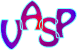VASP Plane-wave PAW code. Used in  spin polarized mode. Requires a specific license. 525 (4.6)QuantumEspresso Density-functional theory, plane waves, and pseudopotentials based code for electronic-structure calculations and materials modeling. Used in spin polarized mode. 87 SSSP: 0.3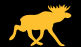ELK Full Potential-All Electrons LAPW code. Used in spin polarized mode. A very efficient all-electrons code. Slow but reliable. 9820 0.3ABINIT Plane-wave pseudopotential code. Can be used with JTH and GBRV pseudo lib (nsteps=100 ecut=1000 eV) 64-87 pps=pawxml (JTH): 0.4  pps=paw (GBRV): 0.8 pps=HGH.k: 2.1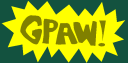GPAW Real-space/plane-wave/LCAO PAW code. 465 1.5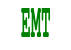EMT Effective Medium Theory calculator (only for Al,Cu,Ag,Au,Ni,Pd,Pt,H,C,N,O). Very fast, but limited. 0.9 Octopus Used in spin polarized mode. Siesta Used in spin polarized mode.
The available calculators in sqw_phonons. We recommend VASP, QuantumEspresso, and Elk. The computation time is given for (Al a=5.6) with 24 cores (2.8GHz), kpoints=4, supercell=3, ecut=default, and 'accurate' setting (using PhonoPy). These times depend on the pseudo potential used, and are only given as estimates.

Benchmarks indicate that, for phonon dispersions:
• Quantum Espresso is the fastest, with excellent parallelization and accuracy.
• ABINIT, VASP and GPAW are also very efficient codes. VASP is solid rock. Results with ABINIT and GPAW may be unstable.
• The all-electrons ELK code is about 10 times slower than QuantumEspresso, but provides reasonable results.
• Using k-points=4 is both fast and ensures a reasonable accuracy in phonons.
• Similarly, a supercell=3 is necessary for small lattice cells, but 2 could be enough for large ones.
• Phonon dispersions are not too sensitive on the energy cut-off. ecut=340 eV is satisfactory. The computational time increases as ecut1.6 for ecut within 200 and 1500 eV, according to our benchmarks.
• Elk can not handle large supercells without recompiling. The Debian package we provide is done so.
• Elk is hardly sensitive to the energy cut-off in results and computational needs.
• NWChem and Dacapo calculators are not supported as they do not provide reliable results for phonon calculations.
• Refer to https://molmod.ugent.be/deltacodesdft for codes comparison. Currently, best codes are QE with SSSP, Elk, Abinit with PAWXML JTH or GBRV, GPAW. VASP 5.2 with PAW2015 GW is also excellent (when available).

Other calculators

It is also possible to use any ASE compliant calculator setting the 'options' fields 'calculator' and 'declaration', as well as the path to the calculator executable in the corresponding ASE command. Use double quotes strings ("...") if needed within the Python expressions.

• options.declaration = 'from ase.calculators.lammpsrun import LAMMPS';
• options.calculator='LAMMPS()';
• setenv('LAMMPS_COMMAND','/usr/bin/lammps');
• s=sqw_phonons('lattice', options)
Options to specify executable locations

It is possible to indicate which executable should be used, rather than using the default system ones.
• options.calculator                Python/ASE calculator (see above)
• options.command='exe'       Path to calculator executable and command line, e.g. 'mpirun -np 24 vasp' to override the default
Single calculator executables can be specified for 'python','mpirun','gpaw','elk','abinit', 'quantumespresso','vasp','octopus','cp2k','siesta', for instance:
• options.python='python3'
which must be used at start-up.
• sqw_phonons('Al.laz', options)
You may also simply use:
• sqw_phonons('Al.laz','python=python3; quantumespress=pw.x')
which can contain full path. To change the configuration after initial use (e.g. move from python 2 to python3), you should first clear the configuration with:
• clear sqw_phonons
• sqw_phonons('Al.laz','python=python2.7; quantumespress=pw.x')

### Configuring the Calculator

Other options, given as strings, or as a structure, allow to customise how to create the supercell and other computational tuning stuff.

An example is:
`	>> s=sqw_phonons(POSCAR,'ABINIT','nsteps=100; occupations=smearing; ecut=1000; pps=paw;');`
More options can be obtained from 'help sqw_phonons':
• target: The 'target=LOCATION' argument can be set to save these files to a defined directory, which must pre-exist. Without specification, a temporary directory will be used.
• supercell: The dimension of the supercell can be given using e.g. the 'supercell=3' (for a 3x3x3 supercell) argument, or 'supercell=[2 3 2]'. Let supercell=0 (default) to set automatically the supercell from the number of atoms in the material cell as (1000/N)^(1/6).
• calculator: the calculator to use, see below.
• potentials: pseudo potential directory to use, e.g. '/usr/share/elk-lapw/species', '/usr/share/abinit/psp', '/usr/share/espresso/pseudo'.
• diagonalization: 'david' or 'cg'. or 'rmm-diis'. The Davidson method is faster than the conjugate-gradient but uses more memory. The RMM-DIIS is even faster.
• autoplot: evaluates the model on QH=0 plane, and plot it.
• mpi: The number of CPU's to use can be set with option e.g 'mpi=4'. This option requires e.g. OpenMPI to be installed, and is only validated on Linux systems (sudo apt-get install openmpi-bin). All codes support MPI. Remember that the memory usage increases linearly with the number of processors involved, so in practice you should have about 2GB per CPU requested. For 16 GB available memory, you can safely use 8 CPUs.
• command: specify the calculator executable which may include e.g. "mpirun -np N" when applicable (and options.mpi should not be set).
• htmlreport: when set to 1, creates a readable report as an HTML document 'sqw_phonons.html' in the target directory. The syntax sqw_phonons(..., 'report', ...) also works.
• optimizer: when set, performs a geometry optimization before computing the forces. This option can be set to  'BFGS', 'LBFGS' (low memory BFGS), 'MDMin' or 'FIRE', as explained in the ASE Structure optimization page. If the input configuration is not close to its equilibrium state, it is worth setting this option. We recommend to set it to BFGS or MDMin (which are fast) in all cases.
• accuracy: The 'fast' choice uses the symmetry operators to lower the number of atom displacements. The force gradient is using central difference (2 displacements +/- on each axis). The 'very fast' option (default) halves the number of displacements and, when available, uses PhonoPy. The equilibrium forces are used to improve the accuracy of the force gradient. The 'accurate' choice is longer to execute (e.g. 3-6 times slower), and computes all forces ignoring symmetry. All these accuracy settings display the ETA before completion, but are limited to 256 displacements. This limit can be reached when computing with large cells. In this case, you may use the 'single_shot' accuracy setting which does all computations in one shot (no ETA is displayed). This is usually a very fast solution as well.
• use_phonopy: when set to 1, or using directly sqw_phonons(..., 'phonopy', ...), the PhonoPy library is used, which results in a 'very fast' calculation (using forward difference force gradient), and all available symmetry operators. This requires PhonoPy to be available. When not available, the default 'very fast' accuracy choice will be used (using PHON methodology).
and more specific DFT related options:
• occupations: For metallic and insulator systems, the electron state occupations should be set by using argument 'metal' (0.3 eV) 'semiconductor' (0.1meV) or 'insulator', or 'occupations=smearing' or 'occupations=fixed'. You can also directly specify the smearing width as 'occupations=<value>' assuming a Fermi Dirac distribution. The unit is eV.
• kpoints: The size of the Monkhorst-Pack grid can be set using e.g. 'kpoints=3' or 'kpoints=[3 4 3]'. In order to compute realistic phonon dispersions, the k-points value should be at least 3. Values lower should only be used for testing. The high accuracy value for kpoints3*supercell3 is 6750/N for a N-atom cell, but you may start with smaller values such as 1000/N. Let kpoints=0 (default) to set automatically the kpoints from the number of atoms in the material cell as ∛(1000/N)/supercell.
• xc: type of Exchange-Correlation functional to use, e.g. default 'xc=PBE'. Other choices 'LDA','revPBE','RPBE',...
• ecut: The kinetic energy cutoff for wavefunctions can also be specified using e.g. argument 'ecut=340'. The unit is eV. A good estimate is to use [200*the number of different atoms]. ABINIT with paw/pawxml potentials requires larger ecut (1000-1500eV) and nsteps (100).
• ecutrho (optional): kinetic energy cutoff for charge density and potential. Typical=4*ecut, suitable for PAW. Larger value improves convergence, especially for ultra-soft PP (you should then use 8-12*ecut). Leave it undefined to let the calculator determine it.
• nsteps (optional): max number of iterations for SCF. Larger value improves convergence. Leave it undefined to let the calculator determine it.
• nbands (optional): Number of valence bands for which wavefunctions are being computed. Should be the number of electrons /2 and for a metal, 20% more (minimum 4 more). Leave it undefined to let the calculator determine it.
• toldfe (optional): Convergence threshold on the energy for selfconsistency. Typical value is 1e-6. Leave it undefined to let the calculator determine it.

#### Specific options for ABINIT

We recommend the PAWXML PBE JTH and PAW PBE GBRV potentials.
• iscf: type of self-consistent cycle. Can be 'NC' (norm-conserving) and 'PAW'.
• potentials: can be a directory path, as well as also one of 'NC' 'fhi' 'hgh' 'hgh.sc' 'hgh.k' 'tm' 'paw' 'pawxml' which is then sent to pps below..
• pps: Type of pseudo potential database, any of
• 'fhi' Fritz-Haber-Institute, fast but not very accurate
• 'hgh' Hartwigsen-Goedeker-Hutter (without semi-core valence electrons) rather fast and moderately accurate
• 'hgh.sc' Hartwigsen-Goedeker-Hutter (with semi-core valence electrons) rather fast and moderately accurate
• 'hgh.k' Hartwigsen-Goedeker-Hutter (high k) rather fast and moderately accurate
• 'tm' Troullier-Martins
• 'paw' Projector-Augmented-Wave from ATOMPAW, very good
• 'pawxml' PAW in XML format (from GPAW) the best, requires more iterations (e.g. ecut=1500; nsteps=100)

#### Specific options for GPAW

• mode: GPAW computation mode as Plane-Wave, Finite Difference, or LCAO (linear combination of atomic orbitals). Can be 'pw','fd', or 'lcao'. Default is 'pw'. The 'lcao' mode is fast for large systems, and requires less memory.

#### Specific options for Octopus

• pps: Type of pseudo potential database, any of
• 'standard' LDA only for H, Li, C, N, O, Na, Si, S, Ti, Se, Cd
• 'hscv_pbe' Hamann-Schlueter-Chiang-Vanderbilt
• 'hscv_lda' Hamann-Schlueter-Chiang-Vanderbilt
• 'hgh_lda' (Hartwigsen-Goedecker-Hutter LDA pseudopotentials for elements from H to Rn)
• 'sg15' (Optimized Norm-Conserving Vanderbilt PBE up to Bi, no Lanthanides)

#### Specific options for Siesta

• potentials: should be defined as a path to a directory containing the pseudo-potentials, e.g. '/usr/share/siesta/pseudo/'
• pps: Type of pseudo potential database, any of
• 'fhi' Fritz-Haber-Institute (from ABINIT).
• 'tm' Troullier-Martins (from NNIN).

#### Specific options for VASP

• pps: can be a structure to define explicitly which flavour of pseudo-potentials have to be used, per element, such as
struct('K','_pv', 'Ca', '_pv', 'Rb', '_pv', 'Sr', '_sv', 'Y',  '_sv', 'Zr', '_sv', 'Nb', '_pv', 'Cs', '_sv', 'Ba', '_sv', 'Fr', '_sv', 'Ra', '_sv', 'Sc', '_sv')
or better struct('B','_h','C','_h','N','_h','O','_h','F','_h','H','_h','Li','_sv','Be','_sv','Na', '_sv','Mg','_pv','K','_sv','Ca','_sv','Rb','_sv','Sr','_sv','Cs','_sv','Ba','_sv','Sc','_sv','Ti','_pv', 'V','_pv','Cr','_pv','Mn','_pv','Fe','_pv','Ni','_pv','Cu','_pv','Y','_sv','Zr','_sv','Nb','_pv','Mo','_pv', 'Tc','_pv','Ru','_pv','Rh','_pv','Pd','_pv','Hf','_pv','Ta','_pv','W','_pv','Re','_pv','Os','_pv','Al','_h', 'Si','_h','P','_h','S','_h','Cl','_h','Ga','_h','Ge','_h','In','_d','Sn','_d','Tl','_d','Pb','_d','Bi','_d','Fr','_sv','Ra','_sv')

### Convergence issues: what to try

It is generally recommended to use the following settings for accurate phonon band computations:

• kpoints=6 (or at least 4x4x4). kpoints=3 is suggested for a quick estimate.
• ecut=1500 [eV]. This depends on the crystal. Try 340 eV and gradually increase value for better accuracy.
• smearing=0.27 [eV] for most materials. This helps convergence.
• toldfe=1e-6 [eV] or lower. The default is 1e-5 for fast estimates.

If the SCF convergence fails, you may try:

`  >> s=sqw_phonons(POSCAR, 'nsteps=200; toldfe=1e-6; occupations=0.27; ecut=1500; ');`
For insulators, you may also use a very small smearing, e.g. 0.01 eV.
If you are tight on the memory (e.g. the system is large), you may try:
`  >> s=sqw_phonons(POSCAR, 'ecut=500; diagonalization=cg');`
If the convergence can not be reached, relax the tolerance criteria on the energy 'toldfe'. However, the default value is already very relaxed (1e-5) and you should rather play with the other parameters.

If the phonon calculation does converge, but the results show many unstable modes, you may try to reduce the toldfe parameter to says 1e-6 or even lower (down to 1e-8).

### Other tools: powder average, density of states, etc.

The thermo-chemistry quantities (U,F,S,Cv) can be obtained with the thermochemistry(s) call.
The vibrational density of states can be obtained with the dos(s) call.
The standard dispersion curve plot along principal crystal directions can be obtained with the band_structure(s) call.

See more details here.

#### Powder average

Once such a 4D S(hkl,w) iFunc model has been built, it is possible to compute the 2D powder average S(|q|,w) using:
`	>> s=sqw_phonons([ ifitpath 'Data/POSCAR_Al'],'metal','EMT');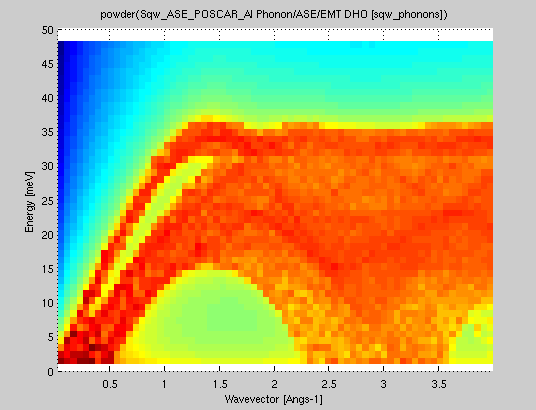>> pow=powder(s); plot(log(pow));`
The same procedure can be applied on an evaluated iData object out of the model.
```	>> qh=linspace(0.01,3,50);qk=qh; ql=qh; w=linspace(0.01,50,51);	>> f=iData(s,[],qh,qk,ql,w);	>> pow=powder(f); plot(log(pow));
```
In both cases, when the reciprocal lattice information is found in the object (matrix B=[a* b* c*]), the conversion from 'rlu' (e.g. 2pi/a units) to 'Angs-1' is automatically done (and this information is stored in the Model upon creation).

### Examples

A usage example is:`	>> s=sqw_phonons('bulk("Cu", "fcc", a=3.6)','EMT','metal'); 	% create model from bulk	>> qh=linspace(0.01,.5,50);qk=qh; ql=qh'; w=linspace(0.01,50,51); 	>> f=iData(s,[],qh,qk,ql,w); 		% evaluate model into an iData	>> plot3(log(f(:,:,1,:)));		% plot ql=0 plane`
To get a system with acoustic and optical modes:
`	>> s=sqw_phonons([ ifitpath 'Data/POSCAR_AlN'],'EMT'); 	% create model from given POSCAR	>> qh=linspace(0.01,.5,50);qk=qh; ql=qh'; w=linspace(0.01,50,51); 	>> f=iData(s,[],qh,qk,ql,w); 		% evaluate model into an iData	>> scatter3(log(f(:,:,1,:)));		% plot ql=0 plane with acoustic and optical modes`
In addition, the phonon Density of States (aka phonon spectrum) is computed, when the model evaluation is requested on the whole Brillouin zone (HKL vary on [-.5 : 0.5]). The results are stored in the model UserData.DOS and UserData.DOS_partials, so that it can be viewed with e.g. :
`	>> s=sqw_phonons([ ifitpath 'Data/POSCAR_Al'],'EMT','report); 	% create model from given POSCAR and report	>> qh=linspace(-0.5,.5,50);qk=qh; ql=qh'; w=linspace(0.01,50,51); 	>> f=iData(s,[],qh,qk,ql,w); 		% evaluate model into an iData, and DOS, U,S,F, Cv 	>> figure; plot(s.UserData.DOS);`
The calculation of the phonon spectrum also provides an estimate of the entropy S, Helmholtz free energy F, internal energy U and the specific heat at constant volume Cv as a function of the temperature. In this example, a URL pointing to the automatically generated report is shown at the end of the procedure (e.g. wait 5 min for completion).

The plot (surf), plot3, scatter3, and slice methods for plotting all provide nice looking rendering of volume data. See Plot/3D page.

### Requirements and installation

Most DFT codes require a multi-core computer with about 4Gb/core memory. Use option e.g. 'mpi=8' to make use of parallelization. When not given and MPI is installed, all processors will be used by default.

Typically, a 16-cores machine allows to run simple material (e.g. binary/ternary materials with up to 10 atoms per cell) phonon estimates within few hours. More complex materials will require e.g. a few days. In terms of memory, you should have 2-3 Gb per core, that is to say 32 Gb RAM. When installed on disk, the whole system requires less that 10 Gb, and you need some limited storage. So, in short 100 Gb is fair.

The ASE must be installed. Packages exist for Ubuntu/Mint/Debian, RedHat/Fedora, OpenSUSE, MacOSX (brew), and Windows (experimental). In addition, the hdf5storage python package must be installed as well. External calculators (e.g. QE, ABINIT, VASP, ...) should be installed separately.

The required software needed to perform Phonon estimates are (see below for the installation procedure):
See below on how to install these packages. If some of the packages are not available, or not functional, iFit-Phonons will still be usable with the other functional parts. It is however recommended to install most of this list.

#### Virtual Machine (our recommendation)

The fastest way to have a running system for Phonon estimates using iFit is to download our ILL/CS Live ready-to-run system with Phonons, McStas and iFit (standalone) all pre-installed.

Install e.g. VirtualBox, then open the OVA file. Set its memory, CPU, etc and import the VM. Click Start. The user account is 'lambda' and its pass word is 'illill'.

#### Debian systems (Ubuntu/Debian/Mint)

For Debian systems, we provide most packages at packages.mccode.org (currently validated on Ubuntu 14.04/Debian 8 and 16.04). To install all in one go, type (Ubuntu/Mint/Debian, replace 'xenial' by 'trusty' for 14.04 and Debian 8 systems):
```% sudo apt-add-repository 'deb http://packages.mccode.org/debian stable main'% sudo apt-add-repository 'deb http://packages.mccode.org/debian xenial main'
% sudo apt-get update% sudo apt-get install ifit-phonons mcrinstaller libxmu6 libxt6 libxp6 libxpm4 libxc1```
which is equivalent to
`% sudo apt-get install ifit elk-lapw gpaw python-matplotlib mcrinstaller libxmu6 libxt6 libxp6 libxpm4% sudo apt-get install abinit abinit-doc abinit-psp quantum-espresso quantum-espresso-sssp phon libnetcdf-dev libxc1 openmpi-bin % sudo apt-get install abinit-psp-jth abinit-psp-gbrv python-ase-qe-spglib python-hdf5storage python-ase python-phonopy`
Note: The gpaw-setups package is named gpaw-data in Ubuntu distributions >= 15.10. Do not install gpaw-setups then.
If some of the packages do not install properly, you can skip them (except iFit and ASE). Then, you will still have access to the other calculators.

The PhonoPy library can be used when installed. To install it, use:
`% sudo apt-get install python-dev python-numpy python-matplotlib python-yaml python-h5py `python-scipy`% sudo pip install phonopy`
or
`% sudo apt-get install python-phonopy (when using our packages.mccode.org repository)`
Python3:
iFit-Phonons can be used with Python3 as well. The required packages to install are then:
• sudo apt install python3-ase python3-hdf5storage
Option: web service. If in addition you wish to use the web interface (which is a very simple access solution without learning how to use the iFit-Phonons software itself), install the package ifit-web-services:
`sudo apt-get install ifit-web-services`
Then open a browser and enter the URL address http://localhost/ifit-web-services . To benefit from the email tracking (start/stop of computations) you will need to edit the file /usr/lib/cgi-bin/computing_sqw_phonons.pl as administrator and change the email server/account/password to use.

#### Other Linux distributions

In principle, it is possible to install the iFit Phonons infrastructure on RedHat class systems (e.g. Fedora), either by installing packages manually, or converting Debian packages into RPM ones using 'alien'.

Use your package manager (e.g. yum and dnf), if available, to install some of e.g.
python-ase, quantum-espresso, elk-lapw, gpaw, abinit.
Then install the standalone linux executable of ifit (standalone, zip archive) from e.g. http://ifit.mccode.org/Downloads/binary/glnx64/ into e.g. /usr/local/ifit. Also install the MCRInstaller in /opt/MATLAB. Create a link to the ifit exeutable with e.g.:
`sudo ln -s /usr/local/ifit/ifit /usr/local/bin/ifit`
You may try to install the ifit-web-services package from https://github.com/McStasMcXtrace/iFit-Web.

#### Windows 10

There are basically 2 recommended installations. The first is via Anaconda (see below).

The second strategy is using the Windows Subsystem for Linux (WSL): Ubuntu / Windows 10

When running Windows 10, you can install a full Linux/Ubuntu subsystem.
Then follow the above instructions for Debian/Ubuntu and get packages from our Debian main repository.
Install then with e.g.:

`# sudo apt-add-repository -y http://packages.mccode.org/debian stable main# sudo update# sudo apt install ifit mcrinstaller libxmu6 libxt6 libxp6 libxpm4 libxc1`
and optionally
`# sudo apt install ifit-phonons`
Then launch iFit (and other commands) from the Linux subsystem 'bash':
`# ifit`

#### Other systems: Anaconda

Install the standalone executable of ifit (standalone, zip archive) from e.g. http://ifit.mccode.org/Install.html
You need Python, Numpy, SciPy and MatPlotLib to be installed. For this, we recommend that you install the Anaconda distribution.

Install ASE, PhonoPy and QE-util using conda or pip in the Python environment (e.g. Anaconda bash), e.g.:
`conda install phonopy hdf5storage ase`
`conda install -c jochym qeutil`
Finally, install the DFT codes referring to their documentation (see 'requirements' above). Some of the packages may not be easily installable on MacOSX or Windows systems.

Then start the Anaconda bash and launch Matlab/iFit from there (type: matlab or ifit at the prompt).

Python3:
iFit-Phonons can be used with Python3 as well. The required packages to install are then:
• python3-ase python3-hdf5storage
• phonopy (optional)

#### Pseudo potentials

The ABINIT code requires to install an additional library of pseudo potentials. We recommand to get it here (ASE) and extract it in /usr/share/abinit/. We provide the abinit-psp (hgh and tmabinit-psp-jth (pawxml) and abinit-psp-gbrv (paw) packages for Debian. You may alternatively install for ABINIT the package abinit-data (which provides a limited set of pseudo-potentials).

The QuantumEspresso code requires to install an additional library of pseudo potentials. We recommend the SSSP library for which we provide the quantum-espresso-sssp Debian package.
WARNING:
the quantum-espresso-data Debian package conflicts with the quantum-espresso-sssp one. It only provides a minimal pseudo-potential list, and is generally less accurate.

E. Farhi - iFit/fit models - Nov. 27, 2018 2.0.2 - back to Main iFit Page